# Pipe Bending Minimum Tangent Length Data Sheet - Intergraph Smart 3D - Reference Data - Hexagon PPM

## Intergraph Smart 3D Piping Reference Data

Language
English
Product
Intergraph Smart 3D
Subproduct
Piping
Search by Category
Reference Data
Smart 3D Version
13

The Minimum Bend-to-Bend Tangent Length Data node (the PipeBendingMinTangentLenData sheet in the Pipe Bending Manufacturability Rules.xls workbook) defines the minimum tangent length data for varies bend types on pipe bending machines.

Pipe Bending Machine Name (Required)

Enter the bending machine code for which you are defining minimum tangent length data. The bending machines are defined in the Pipe Bending Machine Data node in the Catalog task and on the PipeBendingMachineData sheet in the Pipe Bending Machine Name column.

Pipe Bend Type (Required)

Select the pipe bend type for which you want to define minimum tangent length data. Valid bend type codelist values are defined in the AllCodeLists.xls workbook on the PipeBendType sheet in the Codelist Number column.

Codelist

Name

Bend Type

1

Angle Bend <45°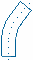2

Angle Bend 45°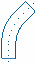3

Angle Bend >45° <90°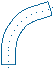4

Quarter Bend5

Offset Bend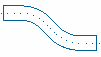6

U-Bend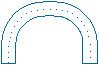7

Angle Bend >90° <180°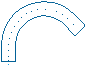8

Single Offset Quarter Bend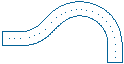9

Crossover Bend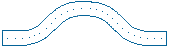10

Single Offset U-Bend11

Expansion U-Bend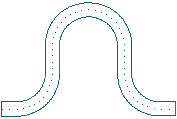12

Double Offset U-Bend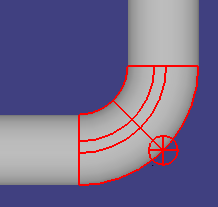13

Double Offset Expansion U-Bend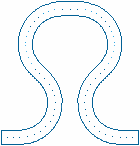Minimum Bend to Bend Tangent Length Option (Optional)

Provides the ability to specify both standard and non-standard minimum bend-to-bend tangent lengths for each type of pipe bend configuration. This option provides alternate minimum tangent lengths on the same bending machine, similar to the commodity option in the piping commodity filter. In this column, enter:

0 to define the row as the standard bend-to-bend tangent lengths

• 1 to define the row as the first set of alternate lengths

• 2 to define the row as the second set of alternate lengths

Nominal Piping Diameter (Required)

Enter the NPD for which to define minimum tangent lengths.

Nominal Piping Diameter UOM (Required)

Specify the units of measurement, for example in or mm, for the Nominal Piping Diameter column.

Type the pipe bend radius multiplier for which the Minimum Bend to Bend Tangent Length Option applies.

Minimum Tangent Length Between Turn 12

Enter the minimum tangent length between turns 1 and 2 that is required for the pipe bending machine. You can enter zero, which represents either a tangent with no length or the non-existent tangent between two turns that are required to represent a single bend, when the nominal bend angle exceeds 90 degrees. This tangent length is used instead of the applicable Minimum Pipe Length value in the piping specification.

Minimum Tangent Length Between Turn 23

Enter the minimum tangent length between turns 2 and 3 that is required for the pipe bending machine. You can enter zero, which represents either a tangent with no length or the non-existent tangent between two turns that are required to represent a single bend, when the nominal bend angle exceeds 90 degrees. This tangent length is used instead of the applicable Minimum Pipe Length value in the piping specification.

Minimum Tangent Length Between Turn 34

Enter the minimum tangent length between turns 3 and 4 that is required for the pipe bending machine. You can enter zero, which represents either a tangent with no length or the non-existent tangent between two turns that are required to represent a single bend, when the nominal bend angle exceeds 90 degrees. This tangent length is used instead of the applicable Minimum Pipe Length value in the piping specification.

Minimum Tangent Length Between Turn 45

Enter the minimum tangent length between turns 4 and 5 that is required for the pipe bending machine. You can enter zero, which represents either a tangent with no length or the non-existent tangent between two turns that are required to represent a single bend, when the nominal bend angle exceeds 90 degrees. This tangent length is used instead of the applicable Minimum Pipe Length value in the piping specification.

Minimum Tangent Length Between Turn 56

Enter the minimum tangent length between turns 5 and 6 that is required for the pipe bending machine. You can enter zero, which represents either a tangent with no length or the non-existent tangent between two turns that are required to represent a single bend, when the nominal bend angle exceeds 90 degrees. This tangent length is used instead of the applicable Minimum Pipe Length value in the piping specification.

Minimum Tangent Length Between Turn 67

Enter the minimum tangent length between turns 6 and 7 that is required for the pipe bending machine. You can enter zero, which represents either a tangent with no length or the non-existent tangent between two turns that are required to represent a single bend, when the nominal bend angle exceeds 90 degrees. This tangent length is used instead of the applicable Minimum Pipe Length value in the piping specification.

Minimum Tangent Length Between Turn 78

Enter the minimum tangent length between turns 7 and 8 that is required for the pipe bending machine. You can enter zero, which represents either a tangent with no length or the non-existent tangent between two turns that are required to represent a single bend, when the nominal bend angle exceeds 90 degrees. This tangent length is used instead of the applicable Minimum Pipe Length value in the piping specification.

Optional Minimum Grip Length (Optional)

Enter the minimum grip length required for the pipe bend configuration. This grip length value will override the value in the pipe bending die data.

Optional Minimum Pull Length (Optional)

Enter the minimum pull length required for the pipe bend configuration. This pull length value will override the value in the pipe bending die data.